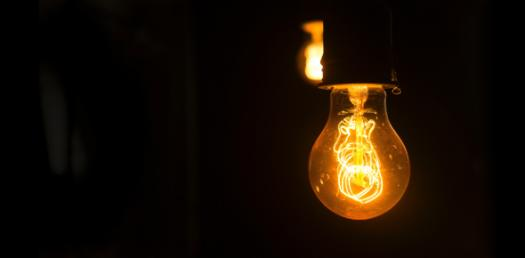# Quiz: Take Some Basic Questions From Electricity!

12 QuestionsSettings.

Related Topics
• 1.
Electricity is the flow of tiny subatomic particles called....
• A.

Electrodes

• B.

Electrons

• C.

Ectrons

• D.

Electrolytes

• 2.
Where in an atom are electrons found?
• A.

In the nucleus (centre)

• B.

Near the middle

• C.

On the top of the nucleus but under the protons

• D.

Whizzing around in the space around the nucleus

• 3.
Which of the following are TRUE for electrons
• A.

Electrons have a negative charge

• B.

Electrons are attracted to other things with a negative charge

• C.

Electrons are attracted to things with a positive charge

• D.

Electrons can never get away from the atom they are originally in

• 4.
If electrons can flow through a substance, we say the material is a conductor.
• A.

True

• B.

False

• 5.
Tick all the things that are INSULATORS (materials that do not allow electricity to flow through them)
• A.

Wood

• B.

Plastic

• C.

Copper wire

• D.

Cloth

• 6.
Batteries, cells and power packs are NOT power sources
• A.

True

• B.

False

• 7.
We call electrical components hooked up by wires so that electrons can flow from and back to a power source a CIRCUIT
• A.

True

• B.

False

• 8.
Which of the following MUST a circuit have?
• A.

Wires and a lamp

• B.

Components and wires

• C.

Wires and a power source and another component

• D.

A battery and a lamp

• 9.
Tick all the options where the substance is a CONDUCTOR (allows electricity to flow through it).
• A.

A lamp

• B.

A copper wire

• C.

Plastic

• D.

A voltmeter

• 10.
Which of the following would be used to measure current (A, amps)?
• A.

A lamp

• B.

An ammeter

• C.

A wire

• D.

A voltmeter

• 11.
What does a voltmeter measure?
• A.

Villige

• B.

Vampage

• C.

Voltage

• D.

Volume of electrons